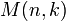# Unipotent element of linear algebraic group

Suppose$G$ is a linear algebraic group over a field$k$, i.e.,$G$ is identified as a closed subgroup of the general linear group$GL(n,k)$. Denote the identity element of$G$ by$1$.
An element$g \in G$ is termed unipotent if$g - 1$ (the subtraction being done with respect to the additive structure of matrices) is a nilpotent element in the matrix ring$M(n,k)$. By degree considerations, this is equivalent to requiring that$(g - 1)^n = 0$, where the powering is done in the matrix ring.